# Regular Grammars Chapter 7 Regular Grammars A regular

• Slides: 11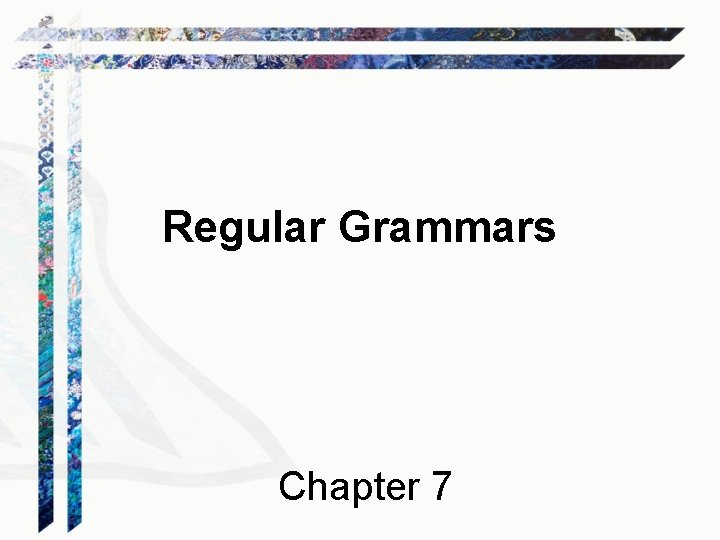Regular Grammars Chapter 7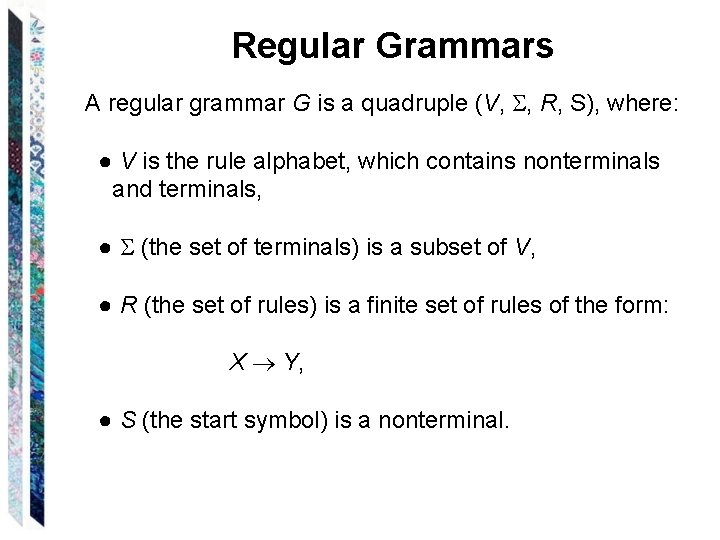Regular Grammars A regular grammar G is a quadruple (V, , R, S), where: ● V is the rule alphabet, which contains nonterminals and terminals, ● (the set of terminals) is a subset of V, ● R (the set of rules) is a finite set of rules of the form: X Y, ● S (the start symbol) is a nonterminal.Regular Grammars In a regular grammar, all rules in R must: ● have a left hand side that is a single nonterminal ● have a right hand side that is: ● , or ● a single terminal followed by a single nonterminal. Legal: S a, S , and T a. S Not legal: S a. Sa and a. Sa T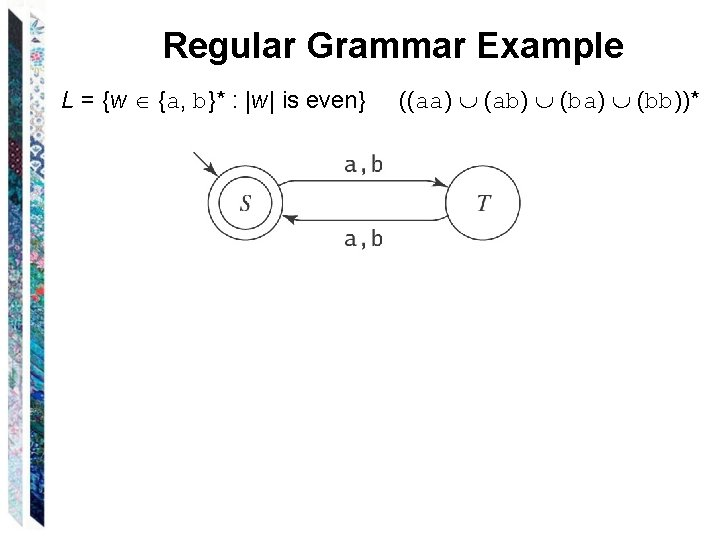Regular Grammar Example L = {w {a, b}* : |w| is even} ((aa) (ab) (ba) (bb))*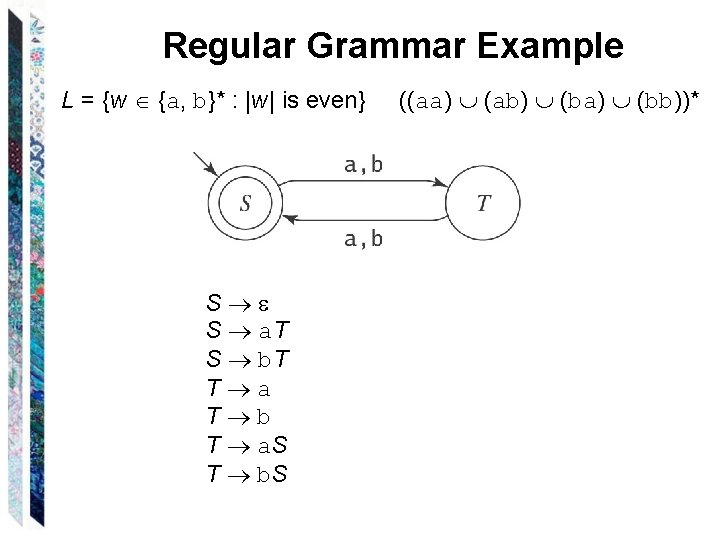Regular Grammar Example L = {w {a, b}* : |w| is even} S S a. T S b. T T a T b T a. S T b. S ((aa) (ab) (ba) (bb))*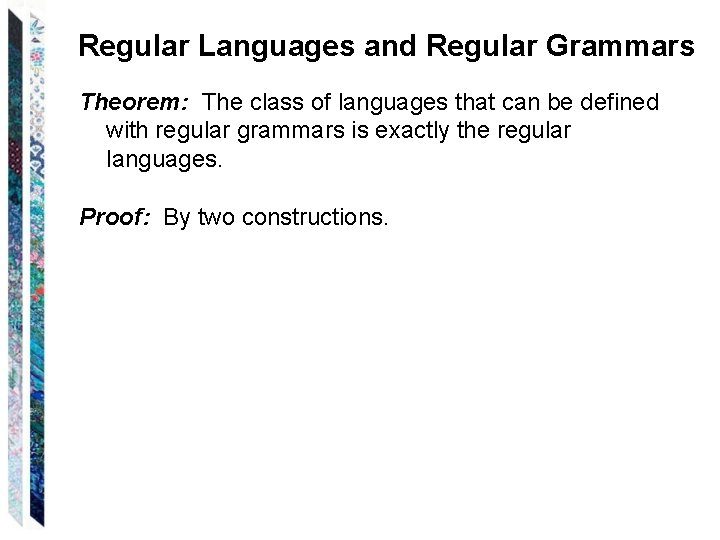Regular Languages and Regular Grammars Theorem: The class of languages that can be defined with regular grammars is exactly the regular languages. Proof: By two constructions.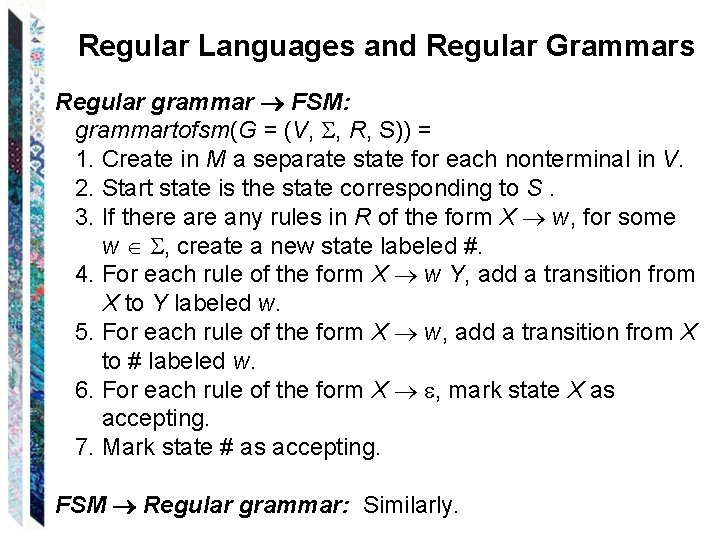Regular Languages and Regular Grammars Regular grammar FSM: grammartofsm(G = (V, , R, S)) = 1. Create in M a separate state for each nonterminal in V. 2. Start state is the state corresponding to S. 3. If there any rules in R of the form X w, for some w , create a new state labeled #. 4. For each rule of the form X w Y, add a transition from X to Y labeled w. 5. For each rule of the form X w, add a transition from X to # labeled w. 6. For each rule of the form X , mark state X as accepting. 7. Mark state # as accepting. FSM Regular grammar: Similarly.Example 1 - Even Length Strings S S a. T S b. T T a T b T a. S T b. SStrings that End with aaaa L = {w {a, b}* : w ends with the pattern aaaa}. S a. S S b. S S a. B B a. C C a. D D aStrings that End with aaaa L = {w {a, b}* : w ends with the pattern aaaa}. S a. S S b. S S a. B B a. C C a. D D a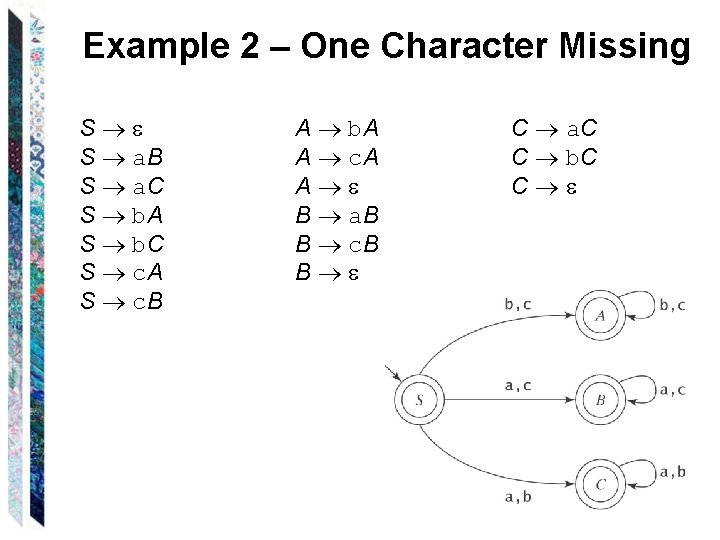Example 2 – One Character Missing S S a. B S a. C S b. A S b. C S c. A S c. B A b. A A c. A A B a. B B c. B B C a. C C b. C C